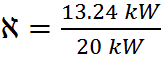# Thermodynamics- determining mechanical power

Gold Member

## Homework Statement

Hello all,

I've worked through the problem below, but I feel my answer is incorrect. I would appreciate it if someone could check my work.

Water is pumped from a lower reservoir to a higher reservoir by a pump that provides 20kW of shaft power. The free surface of the upper reservoir is 45m higher than that of the lower reservoir. If the flow rate of water is measured to be 0.03 m^3/s, determine mechanical power that is converted to thermal energy during this process due to frictional effects.

## Homework Equations

$e_m = ke =(\frac{P}{\rho})(\frac{1}{2}\vec{V}^2)(gz)$

$\dot{E}=\dot{m}e_m$

$\dot{m}=\rho\dot{V}$

$\eta=\frac{energy-out}{energy-in}$

## The Attempt at a Solution

$e_m = ke =(\frac{P}{\rho})(\frac{1}{2}\vec{V}^2)(gz)=gz=(9.81\frac{m}{s^2})(45m)=441.45\frac{m^2}{s^2}=441.45\frac{J}{kg}$

$\dot{m}=\rho\dot{V}=(1 \frac{kg}{m^3})(0.03\frac{m^3}{s})=0.03\frac{kg}{s}$

$\dot{E}=\dot{m}e_m=(0.03\frac{kg}{s})(441.45\frac{J}{kg})=13.24\frac{J}{s}=13.24W = 13.24*10^{-3}kW$

$\eta=\frac{13.24*10^{-3}kW}{20kW}=.66*10^{-3}=0.066$%

$20kW-20\eta= 19.99 kW$

So 19.99 kW out of 20 are wasted to heat in this problem? doesn't seem correct.

Staff Emeritus
Homework Helper
You have the density of water as 1 kg / m^3. This is some really, really light water.

•baldbrain
Gold Member
Ha, how did I miss that? Thanks.

How's the rest of my method look?

Gold Member
2021 Award
You have the wrong value for the density of water. [edit: nevermind, Steam King beat me to it]

Gold Member
Going through it with the proper density, I come up with 6.76 kW lost to heat. Sound about right?

Last edited:
AbbeyC172
Hello! How did you get 6.76 kW? I am with you until you get the .066%. Even with the correct density of water that should just eliminate your 10^ -3.= .662

20 kW- .662

#### Attachments

Mentor
Hello! How did you get 6.76 kW? I am with you until you get the .066%. Even with the correct density of water that should just eliminate your 10^ -3.

View attachment 218603= .662

20 kW- .662